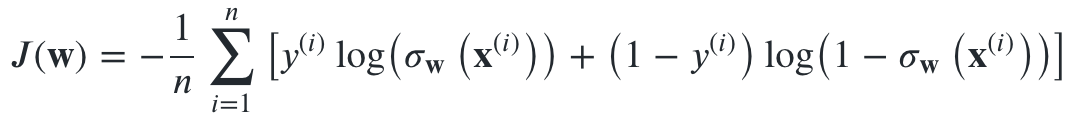# Machine Learning Building Blocks: Logistic Regression

## Logistic Regression from scratch with NumPy.Photo by National Cancer Institute on Unsplash

## Logistic LossLoss function for logistic regression.

An immediate improvement we can make to this is to add regularization, as suggested by Sebastian Raschka and V. Mirjalili in Python Machine Learning. Regularization has the effect of constraining the model by restricting the parameters from becoming too large. In this case, we will use L2 regularization, also known as Ridge Regression when applied to linear and logistic regressions. This will penalise overly large weights in our model, which will hopefully prevent overfitting. This can be implemented by adding the sum of the square of the weights to the end of the cost function, as shown below. J(w) is the cost function we defined above. Lambda is a value we can set, and intuitively Lambda of 0 would remove regularlization, while increasingly large values of Lambda would increase the regularization term of the equation.

We can now put this whole thing together in code for our set of features, labels and weights.

## Intermezzo

Predictably, this did very poorly. But it is good to have something with which to compare our progress!

## Calculating the Gradient

Now all we need is to pick a learning rate, which will be a constant to multiply our gradients by, then increment our weights closer to the minima with each iteration, make new predictions, and repeat.

## Putting it all together

So now that this model is trained, how has it performed? Before training we had an average f1 accuracy of 0.15 on our test data and we have increased that to 0.99. That is probably about as good as we are going to get for this dataset with logistic regression for today.

## Extending Logistic Regression

I’ve written the training and model portions of this tutorial entirely in NumPy to dig out the guts of these methods from low-code frameworks to help any one who wants to gain a deeper understanding of this algorithm. NumPy is a real staple of the Python data science/numerical computing world and is an invaluable tool to learn to be able to implement not only these well known algorithms, but to implement custom algorithms for our own specific use cases. I hope this example helped to shed light on how easy it can be to create these models from scratch and that both technically and conceptually they are very accessible.

## More from Richard Bownes

BBC Data Scientist